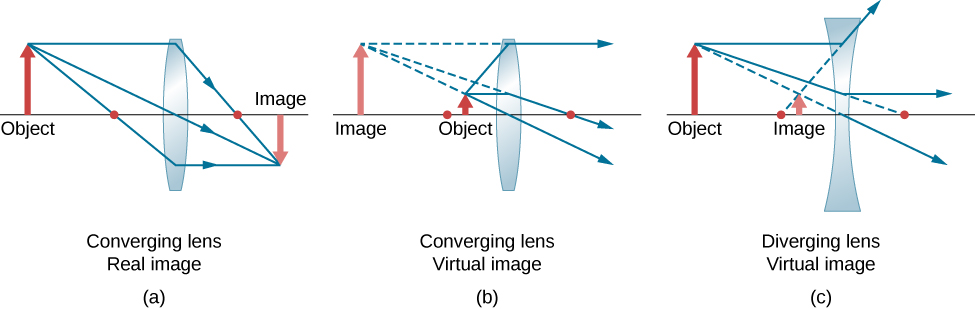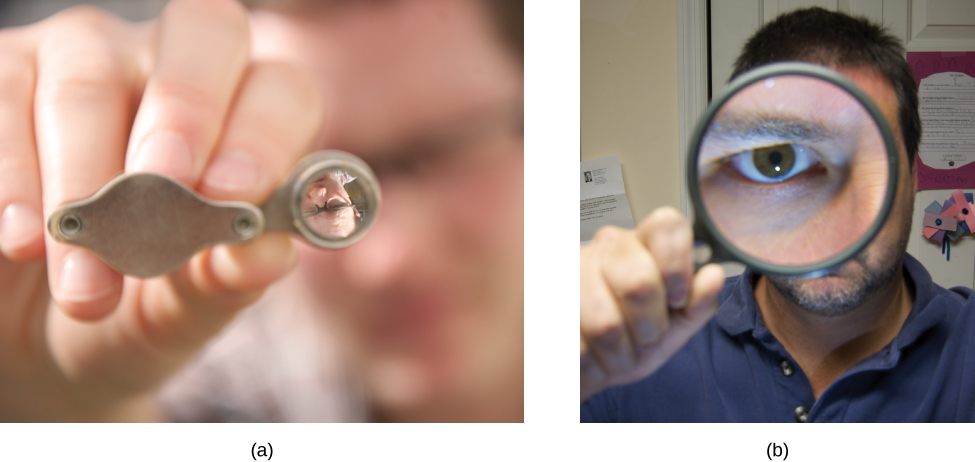# 2.4 Thin lenses  (Page 6/13)

 Page 6 / 13(a) Image distance for a thin converging lens with f = 1.0 cm as a function of object distance. (b) Same thing but for a diverging lens with f = −1.0 cm .

An object much farther than the focal length f from the lens should produce an image near the focal plane, because the second term on the right-hand side of the equation above becomes negligible compared to the first term, so we have ${d}_{\text{i}}\approx f.$ This can be seen in the plot of part (a) of the figure, which shows that the image distance approaches asymptotically the focal length of 1 cm for larger object distances. As the object approaches the focal plane, the image distance diverges to positive infinity. This is expected because an object at the focal plane produces parallel rays that form an image at infinity (i.e., very far from the lens). When the object is farther than the focal length from the lens, the image distance is positive, so the image is real, on the opposite side of the lens from the object, and inverted (because $m=\text{−}{d}_{\text{i}}\text{/}{d}_{\text{o}}$ ). When the object is closer than the focal length from the lens, the image distance becomes negative, which means that the image is virtual, on the same side of the lens as the object, and upright.

For a thin diverging lens of focal length $f=-1.0\phantom{\rule{0.2em}{0ex}}\text{cm}$ , a similar plot of image distance vs. object distance is shown in part (b). In this case, the image distance is negative for all positive object distances, which means that the image is virtual, on the same side of the lens as the object, and upright. These characteristics may also be seen by ray-tracing diagrams (see [link] ).The red dots show the focal points of the lenses. (a) A real, inverted image formed from an object that is farther than the focal length from a converging lens. (b) A virtual, upright image formed from an object that is closer than a focal length from the lens. (c) A virtual, upright image formed from an object that is farther than a focal length from a diverging lens.

To see a concrete example of upright and inverted images, look at [link] , which shows images formed by converging lenses when the object (the person’s face in this case) is place at different distances from the lens. In part (a) of the figure, the person’s face is farther than one focal length from the lens, so the image is inverted. In part (b), the person’s face is closer than one focal length from the lens, so the image is upright.(a) When a converging lens is held farther than one focal length from the man’s face, an inverted image is formed. Note that the image is in focus but the face is not, because the image is much closer to the camera taking this photograph than the face. (b) An upright image of the man’s face is produced when a converging lens is held at less than one focal length from his face. (credit a: modification of work by “DaMongMan”/Flickr; credit b: modification of work by Casey Fleser)

Work through the following examples to better understand how thin lenses work.

## Problem-solving strategy: lenses

Step 1. Determine whether ray tracing, the thin-lens equation, or both would be useful. Even if ray tracing is not used, a careful sketch is always very useful. Write symbols and values on the sketch.

Step 2. Identify what needs to be determined in the problem (identify the unknowns).

Step 3. Make a list of what is given or can be inferred from the problem (identify the knowns).

Step 4. If ray tracing is required, use the ray-tracing rules listed near the beginning of this section.

Step 5. Most quantitative problems require the use of the thin-lens equation and/or the lens maker’s equation. Solve these for the unknowns and insert the given quantities or use both together to find two unknowns.

Step 7. Check to see if the answer is reasonable. Are the signs correct? Is the sketch or ray tracing consistent with the calculation?

what is bohrs model for hydrogen atom
hi
Tr
Hello
Youte
Hi
Nwangwu-ike
hii
Alvin
hi
Siddiquee
what is the value of speed of light
1.79×10_¹⁹ km per hour
Swagatika
what r dwarf planet
what is energy
কাজের একক কী
Jasim
কাজের একক কী
Jasim
friction ka direction Kaise pata karte hai
friction is always in the opposite of the direction of moving object
Punia
A twin paradox in the special theory of relativity arises due to.....? a) asymmetric of time only b) symmetric of time only c) only time
b) symmetric of time only
Swagatika
fundamental note of a vibrating string
every matter made up of particles and particles are also subdivided which are themselves subdivided and so on ,and the basic and smallest smallest smallest division is energy which vibrates to become particles and thats why particles have wave nature
Alvin
what are matter waves? Give some examples
according to de Broglie any matter particles by attaining the higher velocity as compared to light'ill show the wave nature and equation of wave will applicable on it but in practical life people see it is impossible however it is practicaly true and possible while looking at the earth matter at far
Manikant
a centeral part of theory of quantum mechanics example:just like a beam of light or a water wave
Swagatika
Mathematical expression of principle of relativity
given that the velocity v of wave depends on the tension f in the spring, it's length 'I' and it's mass 'm'. derive using dimension the equation of the wave
What is the importance of de-broglie's wavelength?
he related wave to matter
Zahid
at subatomic level wave and matter are associated. this refering to mass energy equivalence
Zahid
it is key of quantum
Manikant
how those weight effect a stable motion at equilibrium
how do I differentiate this equation- A sinwt with respect to t
just use the chain rule : let u =wt , the dy/dt = dy/du × du/dt : wA × cos(wt)
Jerry
I see my message got garbled , anyway use the chain rule with u= wt , etc...
Jerry
de broglie wave equation
vy beautiful equation
chandrasekhar
what is electro statics
when you consider systems consisting of fixed charges
SherlyBy Edgar DelgadoBy OpenStaxBy OpenStaxBy Mahee BooBy Ryan LoweBy Anonymous UserBy OpenStaxBy Rylee MinllicBy Lakeima RobertsBy Brooke Delaney